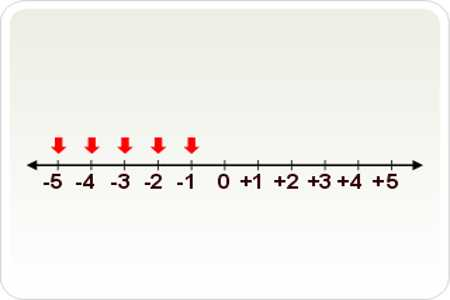Negative Integers are the whole numbers less than zero (i.e. -1, -2, -3, …). This integers have a value less than zero. So, a number which is less than zero but not a fraction or a decimal is called a Negative Integer. The number 0 is simply an integer. It is neither positive nor negative.

Negative Integers Rules

• The negative integers are the inverse of the positive numbers.
• The negative integers are made to have the separate values found in a number line.
• The negative integers are less than zero, denoted as (e.g. -5).
• The zero is neither a positive nor a negative number.
• The number line represents both the positive and negative integers.
• The negative numbers are representing from the left to right.Whether you’re adding positives or negatives, this is the simplest calculation you can do with integers. In both cases, you’re simply calculating the sum of the numbers. For example, if you’re adding two positive integers, it looks like this:

5 + 4 = 9

If you’re calculating the sum of two negative integers, it looks like this:

(- 7) + (- 2) = – 9

To get the sum of a negative and a positive number, use the sign of the larger number and subtract. For example:

(- 7) + 4 = -3

6 + (-9) = – 3

(- 3) + 7 = 4

5 + ( -3) = 2

The sign will be that of the larger number. Remember adding a negative number is the same as subtracting a positive one.

SUBTRACTION

The rules for subtraction are similar to those for addition. If you’ve got two positive integers, you would subtract the smaller number from the larger one. The result will always be a positive integer:

5 – 3 = 2

Likewise, if you were to subtract a positive integer from a negative one, the calculation becomes a matter of addition (with the addition of a negative value):

(- 5) – 3 = -5 + (-3) = -8

If you’re subtracting negatives from positives, the two negatives cancel out and it becomes addition:

5 – (-3) = 5 + 3 = 8

If you’re subtracting a negative from another negative integer, use the sign of the larger number and subtract:

(-5) – (-3) = ( -5) + 3 = -2

(-3) – ( -5) = (-3) + 5 = 2

If you get confused, it often helps to write a positive number in an equation first and then the negative number. This can make it easier to see whether or not a sign change occurs.

MULTIPLICATION

Multiplying integers is fairly simple if you remember the following rule. If both integers are either positive or negative, the total will always be a positive number. For example:

3 x 2 = 6

(-2) x (-8) = 16

However, if you are multiplying a positive integer and a negative one, the result will always be a negative number:

(-3) x 4 = -12

3 x (-4) = -12

If you’re multiplying a larger series of positive and negative numbers, you can add up how many are positive and how many are negative. The final sign will be the one in excess.

DIVISION

As with multiplication, the rules for dividing integers follow the same positive/negative guide. Dividing two negatives or two positives yields a positive number:

12 / 3 = 4

(-12) / (-3) = 4

Dividing one negative integer and one positive integer will result in a negative figure:

(-12) / 3 = -4

12 / (-3) = -4

Conclusion:

All integers (either positive or negative) have a counterpart that is the same distance to zero, but is on the other side of zero. This is one of the characteristics of integers, where we say that all integers have “opposite” counterparts. For example, -7 and +7 are the same distance from zero, so we say that they are opposites of each other. There is no distance between zero and itself, and it’s neither to the left nor the right of itself, so once again we say that zero is neither positive nor negative!

Information Source: